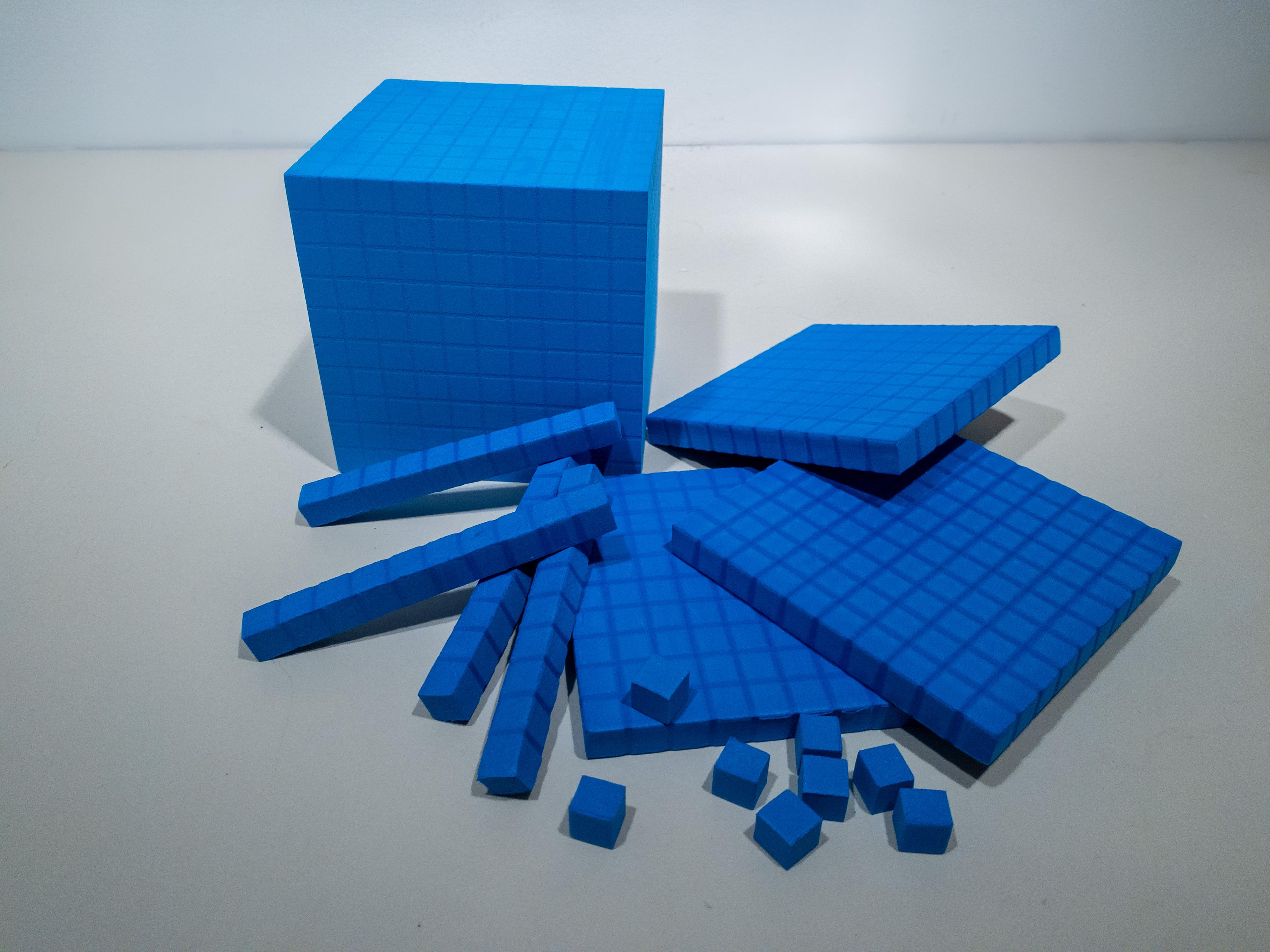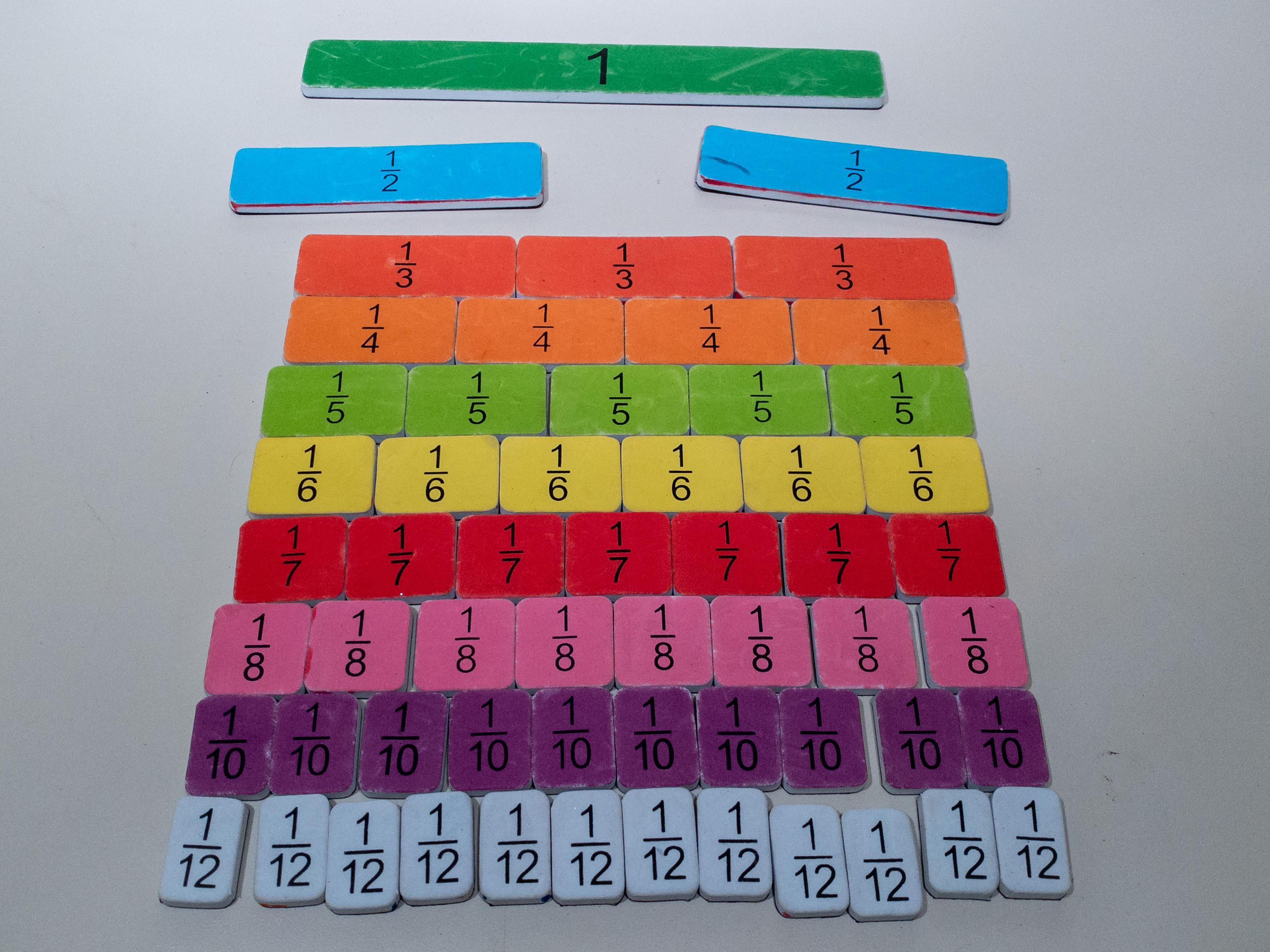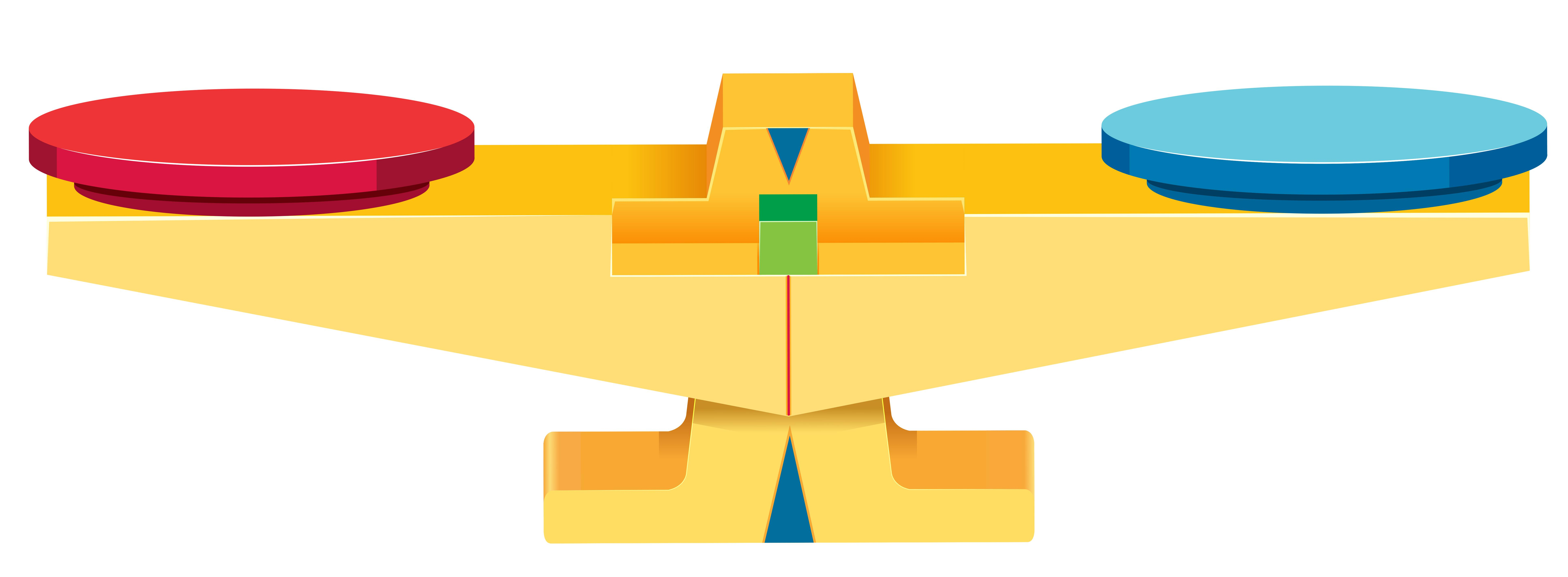All Posts

# Best Math Manipulatives to Have at Home

Aug. 27, 2021

One of the most difficult challenges when teaching math is teaching to the current level of understanding of the student. We take for granted the basics of math. The symbols are a language of their own that we were taught and probably can't remember a time when we didn’t know what to do when we saw a plus sign. Building and reinforcing these fundamentals of math can not be achieved simply with pencil and paper. That is why teachers around the world build libraries of math manipulatives. Manipulatives are physical objects, tools, and demonstrations that take abstract math into the real world. This list provides a guide of the most useful and common manipulatives students will be using in class. These tools can be used to assist with homework, teach lessons or even have students show you what they learned in class. Not to mention simply exploring with these manipulatives will lead students on a journey of mathematical discovery.

Unit Cubes: Frequently called base ten blocks these sets are the backbone to every math curriculum. With a set of unit cubes, teachers and students can demonstrate math as simple as place value positions all the way through the division of decimals. These sets consist of singular cubes which represent ones, straight rods which represent tens, sheets consisting of one hundred cubes representing hundreds and usually one large cube representing one thousand ones. Students will use these cube sets every year to examine increasingly complex math systems. Starting with place value position students can see that ten individual cubes can be aligned to equal one tens rod. Ten rods can be aligned to equal a hundreds sheet. And ten hundreds sheets can be stacked to equal a thousands cube. Cubes can also be used as counters in addition and subtraction, grouped to form multiplication or division, and stacked to learn the basics of rounding. Unit cubes should be the first and most commonly used manipulative in your repertoire.Fraction Tiles: For the first four years of school we tell students one is the smallest number. Our counting system goes from zero to one without pause. Then, without warning, we tell them that there are an infinite amount of fractions in between numbers. Fractions are one of the most abstract parts of elementary mathematics and our word problems about recipes don’t help. What student is baking brownies from scratch? Fraction tiles are a visual representation of what happens when we break one whole into several pieces. Using fraction tiles students can see that greater denominators make smaller pieces, equivalent fractions are the same size and when the number of pieces matches the denominator they get a whole. These lessons can take weeks to teach and still not achieve the fundamental understanding that fraction tiles can.